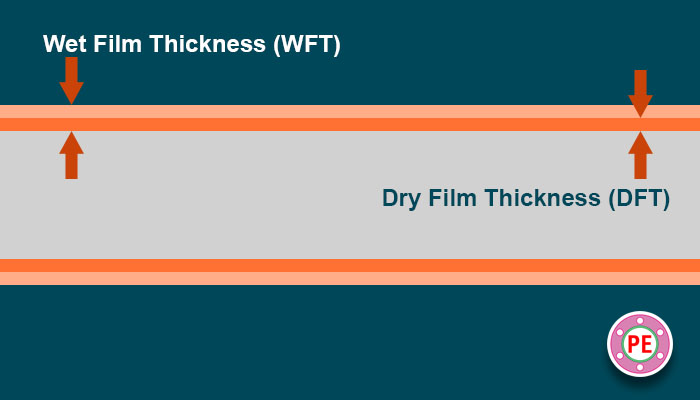# Estimation of Pipe Paint Quantity

## Paint Quantity Estimator

 Pipe NPD : 0.5 0.75 1 1.5 2 3 4 5 6 8 10 12 14 16 18 20 22 24 26 28 30 32 34 36 38 40 42 44 46 48 Required Dry Film Thickness (DFT) Microns : Length of Pipe : MetersFeetMillimeters InchKilometersMiles Percentage of Solids in Paint : Inorganic Zinc Silicate CoatingEtch PrimerZinc Phosphate Epoxy Primer Polyamide Cured High Build Epoxy Micaceous PrimerAcrylic Polyurethan Finish Paint Percentage Loss While Painting : Brush or Roller PaintingAirless Spray PaintingConventional Spray Painting

## Explanation

Required paint quantity for painting of a pipe depends on following factors :

1. Pipe Diameter.
2. Length of Pipe.
3. Required Dry Film Thickness (DFT).
4. Percentage of Solids content in a given paint.
5. Wastage of paint during painting which is based on type of painting method used.

### Wet Paint Thickness

When paint is applied to pipe, it is in wet condition with volatile organic compounds. When it dries, all volatile components goes away leaving only solids component behind. So to achieve particular dry film thickness, higher thickness of wet film is required is is calculated as :

Wet Film Thickness (WFT) = DFT/Fraction of Solids.### Theoretical Paint Quantity

Theoretical Paint quantity is calculated by multiplying total surface area to be painted with wet film thickness.

Theoretical Paint Quantity = Surface Area X WFT.

### Wastage

During painting process, some amount of paint gets wasted which depends on method of paint application. Typical wastage percentages are :

1. Brush or Roller Painting : 10%.
2. Airless Spray Painting : 20%.
3. Conventional Spray Painting : 30%

So, we have to add wastage to calculated paint quantity to get actual paint quantity.

Actual Paint Quantity = Theoretical Paint Quantity / Fraction of Solids in Paint.

## Example Calculation

Pipe of nominal pipe diameter 10″ to be painted with Inorganic Zinc Silicate Coating with solids percentage of 60%. Required dry film thickness is 70 microns. Method of application is conventional Spray Painting. Calculate amount of paint required for length of 100 meters.

1. Pipe Dia in mm (D) = 10″ X 25.4 = 254mm.
2. Length of Pipe (L) = 100 meters = 100000 mm.
3. Surface area of pipe for one meter (A) = Pi X D = 3.141 X 254 = 0.797814 Sq. MM. (It is not necessary to take actual pipe outside diameter from pipe data tables. Difference is negligible.)
4. Total Surface Area = A X L = 797.814 X 10000 0= 79781400 Sq.MM.
5. Required Dry Film Thickness (DFT) = 70 microns = 0.07 mm.
6. Fraction of Solids in Paint (S) = Solids Percent/100 = 60/100 = 0.6
7. Welt Film Thickness (WFT) = (DFT/S) = 0.07/0.6 = 0.1167 mm.
8. Theoretical volume of paint required = Total Surface Area X WFT = 79781400 X 0.1167 = 9310489 Cubic MM. = 9.310489 Liters.
9. For conventional spray painting method, estimated wasted is around 30%. So only 70% paint gets used for actual painting. So amount of actual paint required is theoretical paint quantity divided by fraction of actual paint usage.
10. Actual Paint Volume = Theoretical Paint Volume/0.7 = 9.310489/0.7 = 13.3 Liters.